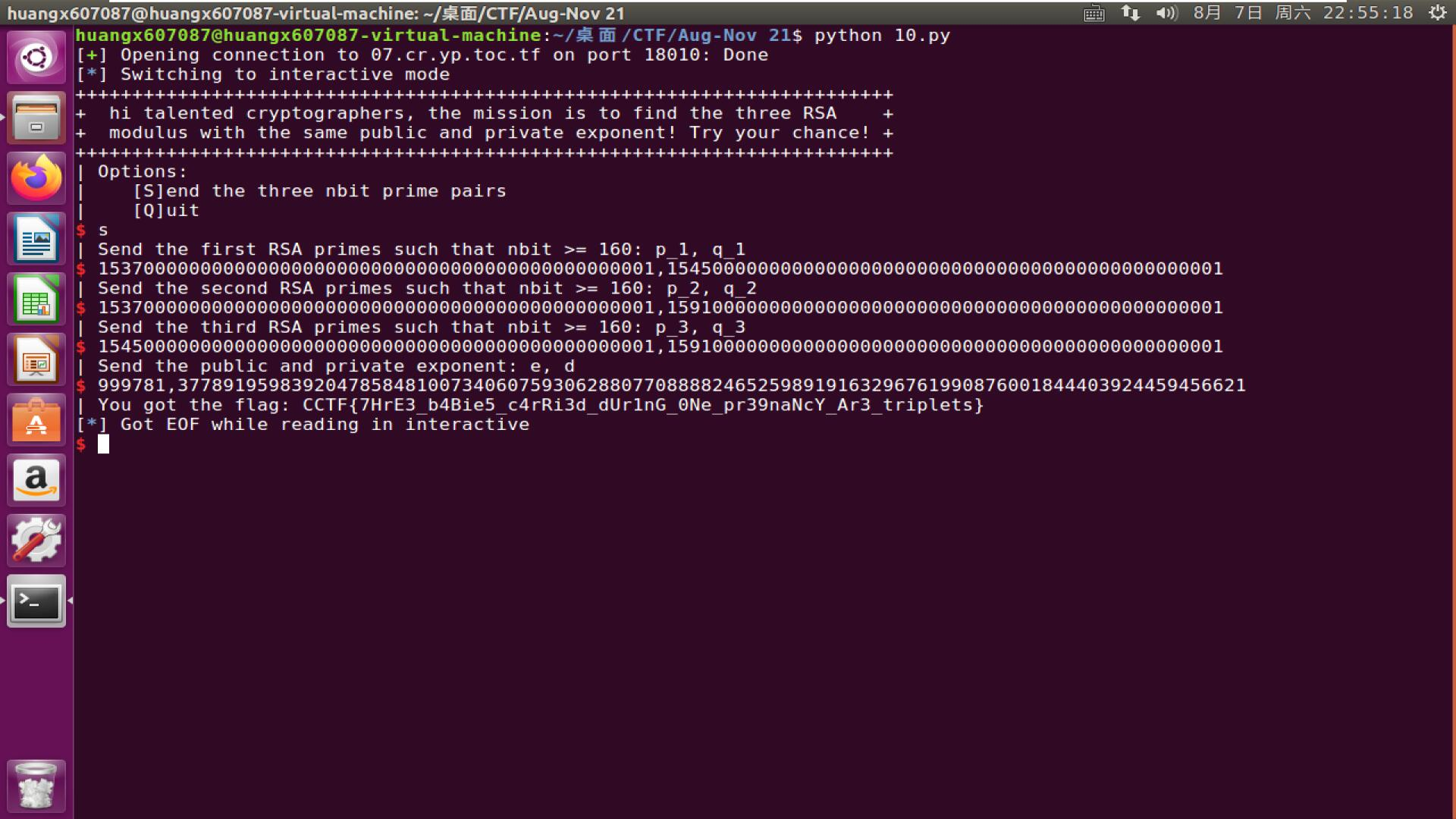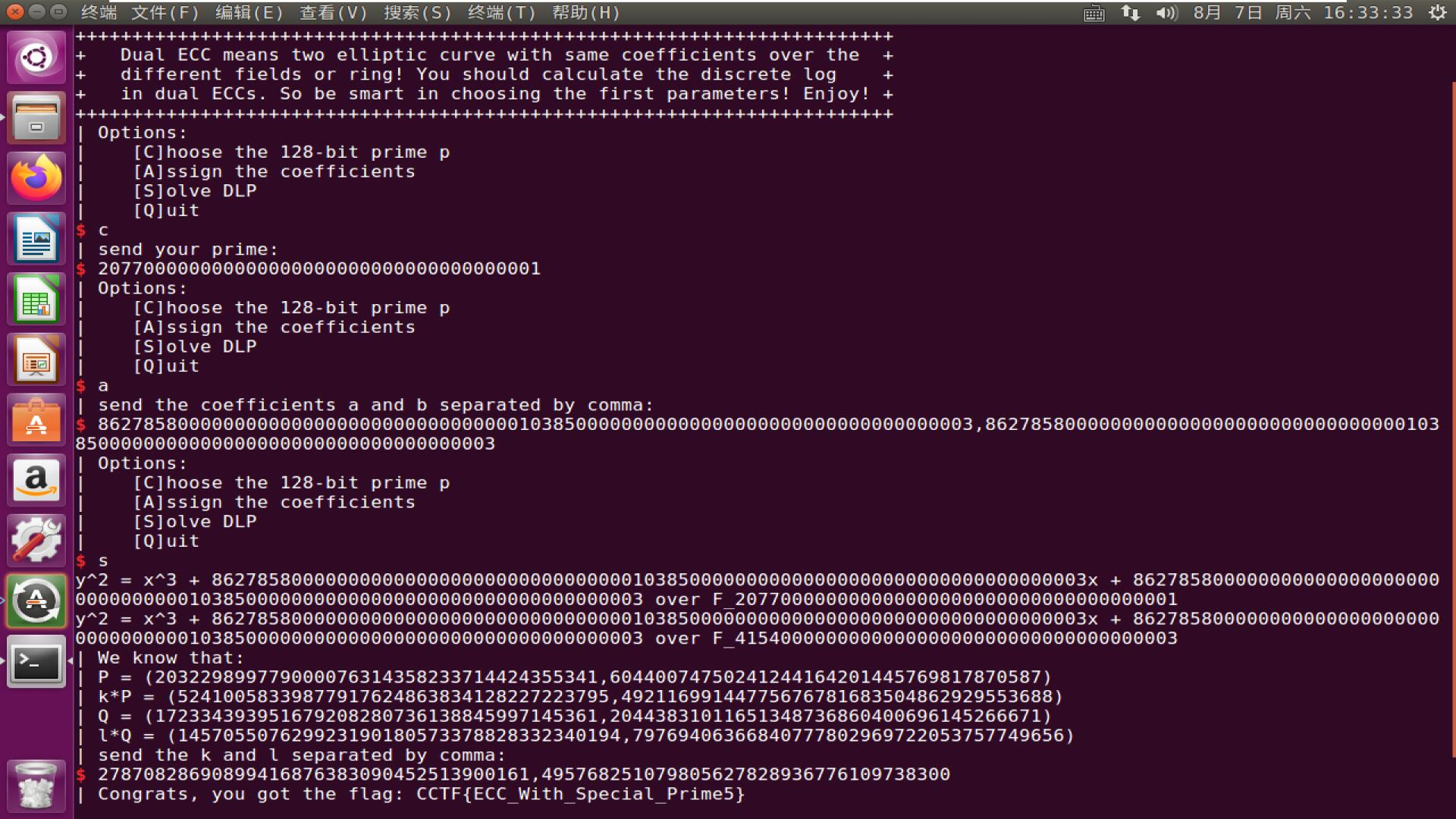# CryptoCTF WriteUp 2 By huangx607087

## 3.Onlude

$$M_3=M_1M_2^{-1}=SLL^{-1}S^{-2}L=S^{-1}L=U^{-1}L^{-1}L=U^{-1}$$

$$M_1M_3^{-1}=SLL^{-1}S=S^2$$

$$R=(M_{10}(S^{-8}))^{-1}=S^8M_{10}^{-1}$$

## 4.Triplet

，这样子三个$\phi$的公约数就很大了。然后我们分别对服务器发送$p,q$，$p,r$，$q,r$，完成第一步。

$$L=3778092015000000000000000000000000000000000000000000000000000000000000000000000000000000000000000001$$

$$31×32251×3778919598392047858481007340607593062880770888824652598919163296761990876001844403924459456621$$## 5.Tiny ECC

$$n=86278580000000000000000000000000000001038500000000000000000000000000000000003$$

$$k=\dfrac {f(Q)}{f(P)}$$目录